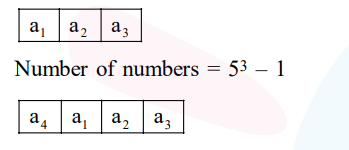# The number of natural numbers less than 7,000`
Question:

The number of natural numbers less than 7,000 which can be formed by using the digits $0,1,3,7,9$ (repitition of digits allowed) is equal to :

1. 250

2. 374

3. 372

4. 375

Correct Option: , 2

Solution:2 ways for $\mathrm{a}_{4}$

Number of numbers $=2 \times 5^{3}$

Required number $=5^{3}+2 \times 5^{3}-1$

$=374$